## Example Questions

← Previous 1 3

### Example Question #1 : Logarithms

Let log 5 = 0.69897 and log 2 = 0.30103.  Solve log 50

1.39794

1.69897

1.36903

1.68794

1.30103

1.69897

Explanation:

Using properties of logs:

log (xy) = log x + log y

log (xn) = n log x

log 10 = 1

So log 50 = log (10 * 5) = log 10 + log 5 = 1 + 0.69897 = 1.69897

### Example Question #2 : Logarithms

y = 2x

If y = 3, approximately what is x?

Round to 4 decimal places.

1.5850

0.6309

2.0000

1.8580

1.3454

1.5850

Explanation:

To solve, we use logarithms. We log both sides and get: log3 = log2x

which can be rewritten as log3 = xlog2

Then we solve for x: x = log 3/log 2 = 1.5850 . . .

### Example Question #3 : Logarithms

Evaluate

log327

9

3

27

30

10

3

Explanation:

You can change the form to

3x = 27

= 3

### Example Question #4 : Logarithms

If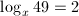, what is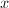?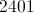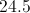Explanation:

If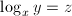, then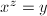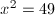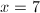### Example Question #5 : Logarithms

If log4  x = 2, what is the square root of x?

12

3

16

4

2

4

Explanation:

Given log4= 2, we can determine that 4 to the second power is x; therefore the square root of x is 4.

### Example Question #6 : Logarithms

Solve for x in the following equation:

log224 - log23 = logx27

2

1

2

9

3

3

Explanation:

Since the two logarithmic expressions on the left side of the equation have the same base, you can use the quotient rule to re-express them as the following:

log224  log23 = log2(24/3) = log28 = 3

Therefore we have the following equivalent expressions, from which it can be deduced that x = 3.

logx27 = 3

x3 = 27

### Example Question #1 : How To Find A Logarithm

What value ofsatisfies the equation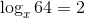?Explanation:

The answer is.can by rewritten as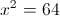.

In this form the question becomes a simple exponent problem. The answer isbecause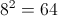.

### Example Question #8 : Logarithms

If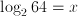, what is?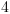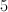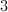Explanation:

Use the following equation to easily manipulate all similar logs: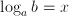changes to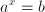.

Therefore,changes to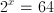.

2 raised to the power of 6 yields 64, somust equal 6. If finding the 6 was difficult from the formula, simply keep multiplying 2 by itself until you reach 64.

### Example Question #9 : Logarithms

Which of the following is a value ofthat satisfies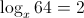?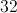Explanation:

The general equation of a logarithm is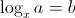, and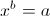In this case,, and thus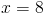(or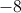, butis not an answer choice)

### Example Question #10 : Logarithms

How can we simplify this expression below into a single logarithm?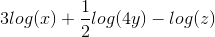Cannot be simplified into a single logarithm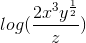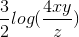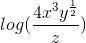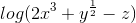Explanation:

Using the property that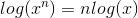, we can simplify the expression to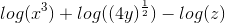.

Given that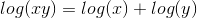and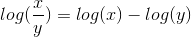We can further simplify this equation to← Previous 1 3